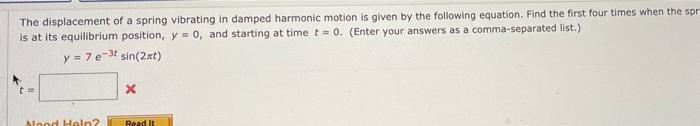Question:

# The displacement of a spring vibrating in damped harmonic motion is given by the following equation. Find the first four times when the spring is at its equilibrium position, y = 0, and starting at tiThe displacement of a spring vibrating in damped harmonic motion is given by the following equation. Find the first four times when the spring is at its equilibrium position, y = 0, and starting at time t = 0.